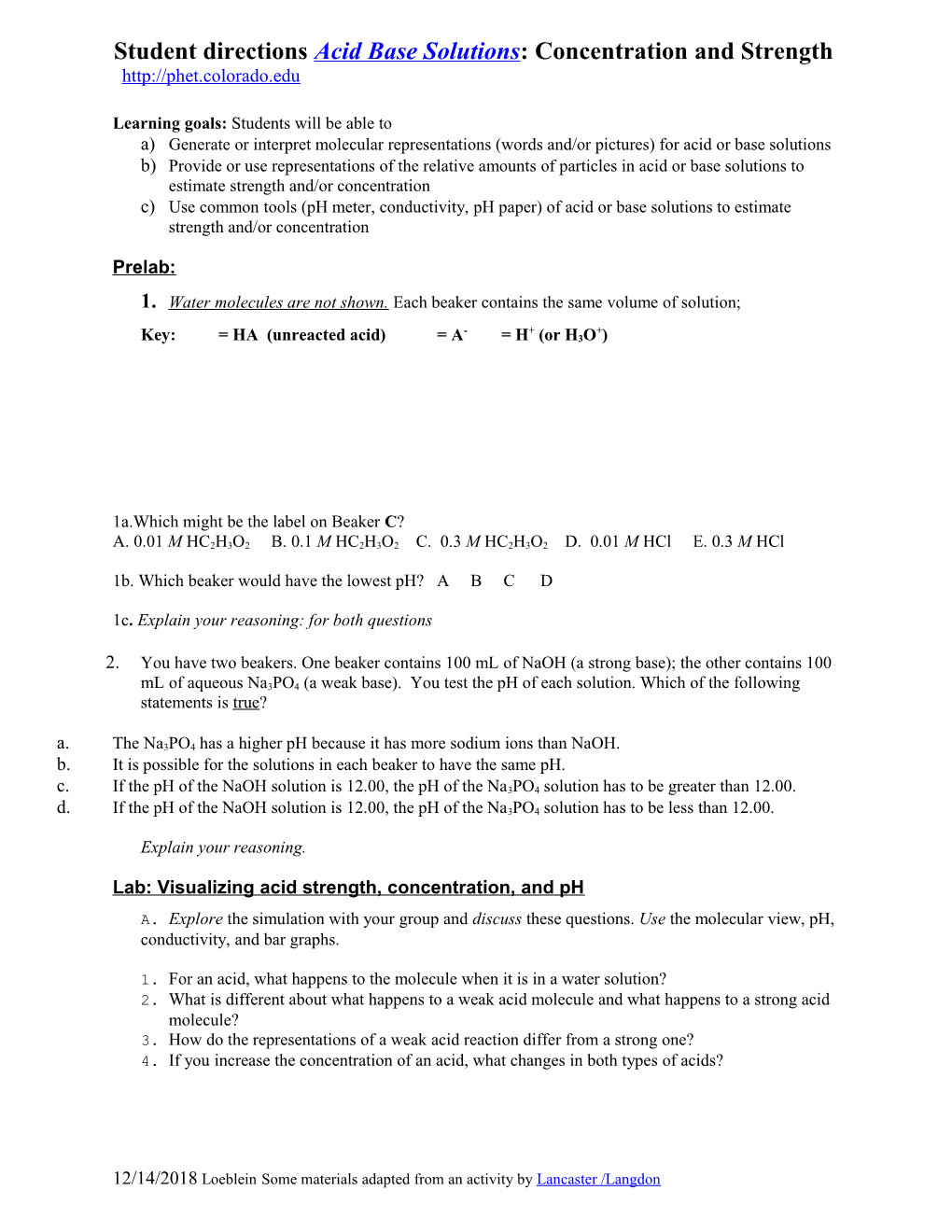# Student Directions Acid Base Solutions : Concentration and StrengthStudent directions Acid Base Solutions: Concentration and Strength

Learning goals: Students will be able to

a)Generate or interpret molecular representations (words and/or pictures) for acid or base solutions

b)Provide or use representations of the relative amounts of particles in acid or base solutions to estimate strength and/or concentration

c)Use common tools (pH meter, conductivity, pH paper) of acid or base solutions to estimate strength and/or concentration

#### Key: = HA (unreacted acid) = A- = H+ (or H3O+)

1a.Which might be the label on Beaker C?

A. 0.01 M HC2H3O2 B. 0.1 M HC2H3O2 C. 0.3 M HC2H3O2 D. 0.01 MHCl E. 0.3 MHCl

1b.Whichbeaker would have the lowest pH? A B C D

1c. Explain your reasoning: for both questions

1. You have two beakers. One beaker contains 100 mL of NaOH (a strong base); the other contains 100 mL of aqueous Na3PO4 (a weak base). You test the pH of each solution. Which of the following statements is true?
1. The Na3PO4 has a higher pH because it has more sodium ions than NaOH.
2. It is possible for the solutions in each beaker to have the same pH.
3. If the pH of the NaOH solution is 12.00, the pH of the Na3PO4 solution has to be greater than 12.00.
4. If the pH of the NaOH solution is 12.00, the pH of the Na3PO4 solution has to be less than 12.00.

#### Lab: Visualizing acid strength, concentration, and pH

1. Explore the simulation with your group and discuss these questions.Use the molecular view, pH, conductivity,and bar graphs.
1. For an acid, what happens to the molecule when it is in a water solution?
2. What is different about what happens to a weak acid molecule and what happens to a strong acid molecule?
3. How do the representations of a weak acid reaction differ from a strong one?
4. If you increase the concentration of an acid, what changes in both types of acids?
1. These images (molecular view of solution/graph/pH/conductivity) depict a strong acid solution:

KEY:1. How does the Key change for a weak acid?
2. How would the images change for a weak acid solution of the same concentration? Draw the images as well as describing them in words.
3. Draw the images for a weak acid and a strong acid solution of the greater concentration? Make any notes that might help you remember.
4. Write the chemical reactions for a weak acid and a strong acid.
5. Is there one type of representation that might be the best one for you to remember information about weak and strong acids? Make any notes that might help you remember how to compare/contrast the two types.
1. Repeat parts A and B for base solutions.
1. If your lab partner explains to you that concentration and strength effect acid base solution representations the same way, are they right? Make notes of ideas that support the statement and those that contradict.

12/31/2018 LoebleinSome materials adapted from an activity by Lancaster /Langdon